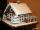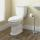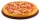# Fraction calculator

The calculator performs basic and advanced operations with fractions, expressions with fractions combined with integers, decimals, and mixed numbers. It also shows detailed step-by-step information about the fraction calculation procedure. Solve problems with two, three, or more fractions and numbers in one expression.

## Result:

### 42/5 - 33/4 = 13/20 = 0.65

Spelled result in words is thirteen twentieths.

### How do you solve fractions step by step?

1. Conversion a mixed number 4 2/5 to a improper fraction: 4 2/5 = 4 2/5 = 4 · 5 + 2/5 = 20 + 2/5 = 22/5

To find new numerator:
a) Multiply the whole number 4 by the denominator 5. Whole number 4 equally 4 * 5/5 = 20/5
b) Add the answer from previous step 20 to the numerator 2. New numerator is 20 + 2 = 22
c) Write a previous answer (new numerator 22) over the denominator 5.

Four and two fifths is twenty-two fifths
2. Conversion a mixed number 3 3/4 to a improper fraction: 3 3/4 = 3 3/4 = 3 · 4 + 3/4 = 12 + 3/4 = 15/4

To find new numerator:
a) Multiply the whole number 3 by the denominator 4. Whole number 3 equally 3 * 4/4 = 12/4
b) Add the answer from previous step 12 to the numerator 3. New numerator is 12 + 3 = 15
c) Write a previous answer (new numerator 15) over the denominator 4.

Three and three quarters is fifteen quarters
3. Subtract: 22/5 - 15/4 = 22 · 4/5 · 4 - 15 · 5/4 · 5 = 88/20 - 75/20 = 88 - 75/20 = 13/20
For adding, subtracting, and comparing fractions, it is suitable to adjust both fractions to a common (equal, identical) denominator. The common denominator you can calculate as the least common multiple of both denominators - LCM(5, 4) = 20. In practice, it is enough to find the common denominator (not necessarily the lowest) by multiplying the denominators: 5 × 4 = 20. In the next intermediate step, the fraction result cannot be further simplified by canceling.
In words - twenty-two fifths minus fifteen quarters = thirteen twentieths.

#### Rules for expressions with fractions:

Fractions - use the slash “/” between the numerator and denominator, i.e., for five-hundredths, enter 5/100. If you are using mixed numbers, be sure to leave a single space between the whole and fraction part.
The slash separates the numerator (number above a fraction line) and denominator (number below).

Mixed numerals (mixed fractions or mixed numbers) write as non-zero integer separated by one space and fraction i.e., 1 2/3 (having the same sign). An example of a negative mixed fraction: -5 1/2.
Because slash is both signs for fraction line and division, we recommended use colon (:) as the operator of division fractions i.e., 1/2 : 3.

Decimals (decimal numbers) enter with a decimal point . and they are automatically converted to fractions - i.e. 1.45.

The colon : and slash / is the symbol of division. Can be used to divide mixed numbers 1 2/3 : 4 3/8 or can be used for write complex fractions i.e. 1/2 : 1/3.
An asterisk * or × is the symbol for multiplication.
Plus + is addition, minus sign - is subtraction and ()[] is mathematical parentheses.
The exponentiation/power symbol is ^ - for example: (7/8-4/5)^2 = (7/8-4/5)2

#### Examples:

subtracting fractions: 2/3 - 1/2
multiplying fractions: 7/8 * 3/9
dividing Fractions: 1/2 : 3/4
exponentiation of fraction: 3/5^3
fractional exponents: 16 ^ 1/2
adding fractions and mixed numbers: 8/5 + 6 2/7
dividing integer and fraction: 5 ÷ 1/2
complex fractions: 5/8 : 2 2/3
decimal to fraction: 0.625
Fraction to Decimal: 1/4
Fraction to Percent: 1/8 %
comparing fractions: 1/4 2/3
multiplying a fraction by a whole number: 6 * 3/4
square root of a fraction: sqrt(1/16)
reducing or simplifying the fraction (simplification) - dividing the numerator and denominator of a fraction by the same non-zero number - equivalent fraction: 4/22
expression with brackets: 1/3 * (1/2 - 3 3/8)
compound fraction: 3/4 of 5/7
fractions multiple: 2/3 of 3/5
divide to find the quotient: 3/5 ÷ 2/3

The calculator follows well-known rules for order of operations. The most common mnemonics for remembering this order of operations are:
PEMDAS - Parentheses, Exponents, Multiplication, Division, Addition, Subtraction.
BEDMAS - Brackets, Exponents, Division, Multiplication, Addition, Subtraction
BODMAS - Brackets, Of or Order, Division, Multiplication, Addition, Subtraction.
GEMDAS - Grouping Symbols - brackets (){}, Exponents, Multiplication, Division, Addition, Subtraction.
Be careful, always do multiplication and division before addition and subtraction. Some operators (+ and -) and (* and /) has the same priority and then must evaluate from left to right.

## Fractions in word problems:Janka and Marienka calculated that there are 210 gingerbreads on the gingerbread house. Janko ate one-seventh of all gingerbreads, and Marienka ate a third less than Janko. How many gingerbreads remained in the gingerbread house?
• ToiletsFederal law requires that all residential toilets sold in the United States use no more than 1 3/5 gallons of water per flush. Before this legislation, conventional toilets used 3 2/5 gallons of water per flush. Find the amount of water saved in one year
• KarinaKarina has 5 4/7 meters of cloth. She needs 3 1/6 meters of it in making a curtain for her window. How many meters of cloth were left to her after making the curtain?
• Metal rodYou have a metal rod that’s 51/64 inches long. The rod needs to be trimmed. You cut 1/64 inches from one end and 1/32 inches from the other end. Next, you cut the rod into 6 equal pieces. What will be the final length of each piece?
• Magic bagEach time the prince crossed the bridge, the number of tolars in the magic bag doubled. But then the devil always conjured 300 tolars for him. When this happened for the third time, the prince had twice as much as he had in the beginning. How many tolars
• Cups of punchCyka made 6 19/20 cups of punch punch at two different types of juice in it. If the punch had 4 1/5 cups of one type of juice how many cups of the other type of juice did it have?
• PizzaThree siblings ordered one pizza. Miška ate a quarter of the whole pizza. Lenka ate a third of the rest and Patrik ate half of what Lenka had left. They had the rest packed up. How much of the pizza did they pack? Write the result as a fraction.
• The petAnanya has a bunny. She bought 4 7/8 pounds of carrots. She fed her bunny 1 1/4 pounds of carrots the first week. She fed her bunny 5/6 pounds of carrots the second week. All together, how many pounds of carrots did she feed her bunny? 1. Draw a tape diag
• KevinKevin is baking brownies and needs peanuts.  The recipe calls for 1 and 2/3 cups of peanuts.  Kevin only has 5/6 of a cup of peanuts.  How many more cups of peanuts does Kevin need to have the correct amount?
• 5 2/55 2/5 hours a week  mathematics,  3 3/4 hours a week   Natural sciences, 4 3/8 hours a week  Technology . how many hours does  he spend on social sciences if he spend 17 1/2 hours a week for the four subject?
• Wood 11Father has 12 1/5 meters long wood. Then I cut the wood into two pieces. One part is 7 3/5 meters long. Calculate the length of the other wood?Sadie practiced her spelling words for 3/4 of an hour, and Max practiced his spelling words for 5/12 of an hour. In the simplest form, how much longer did Sadie practice than Max?Billy ate 1 1/4 pizzas and John ate 1 2/3 pizzas. How much more pizza did John eat than Billy?